###### Descriptions and Examples for the POV-Ray Raytracerby Friedrich A. Lohmueller
Deutsch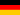Home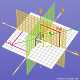- POV-Ray Tutorials

> Analytical Geometry
with POV-Ray
- Index -
- Insert Menu Add-on
& Download

- Basics
Possibilities and Needs

Points & Lines
- Points in 3D
- Line Sections, Straight,
Vectors, Distance Markers
- Surfaces & Planes
- Output of Results,
Captions
- Points of Intersection
- Circles
Solids
- Tetrahedron
- Parallelepiped
- Round Solids
-
- Overview by Table
on "analytical_g.inc"
- Vector Analysis
with POV-Ray
- Righthanded & Lefthanded
Systems of Coordinates
and the Cross Product

- Samples from
Analytical Geometry
- Parallelogram of the
Middles of the Edges
- Trace Points of a Straight Line
- Calculations about a Triangle
- Area of a Parallelogram
and Cross Product
- Shadow of a Pyramid
- Hit a plane || yz-plane
- Angle triangle yz-plane

# Analytical Geometry with POV-Ray Visualizations and Calculations for the Analytical Geometry in 3d Systems of Coordinates with POV-Ray. (Uses Javascript for presentation of a higher resolution of most sample images) update: 08-Feb-2014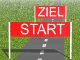0 Basics - What's possible and what you need! An overview on what is possible and what it needs. Basic sample files and include file "analytical_g.inc"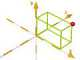1 Points in a 3D System of Coordinates Methods for unequivocal visualization of points in a 3d system of coordinates.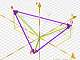2 Line Sections, Straight Lines, Vectors, Distance Markers The representation of line sections, straight lines, vectors, and distance markers.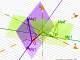3 Surfaces and Planes Triangles, parallelograms, planes by parameters and plane by normalvector.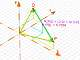4.1 Output of Results and Captions How to make an output of the calculated results and how to add a caption to objects.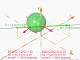4.2 Calculating Points of Intersection How to calculate points of intersections between straight lines and planes or other objects and print the results in the image.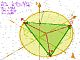5 Circles Circle, circle inside a triangle, circumcircle - circle around a triangle.6.1 Solids I - Tetrahedron Tetrahedron defined by 4 corners, tetrahedron defined by a starting point and 3 vectors.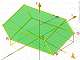6.2 Solids II - Parallelepiped Parallelepiped defined by the corners, parallelepiped defined by a starting point and 3 vectors.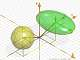6.3 Solids III - Round Solids Sphere, ellipsoid, cylinder, cone.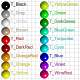7 An Overview by Table The most important commands of POV-Ray and of my include file "analytical_g.pov" and a list of the colors of my template file for a quick orientation.8 Vector Analysis with POV-Ray Table of most important spellings, arithmetic rules and expressions of vector analysis and vector functions in POV-Ray.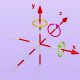9 Righthanded and Lefthanded Systems of Coordinates and the Cross Product Basics on the handyness of systems of coordinates 10 Samples for the Analytical Geometry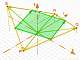10.1 Parallelogram of the Middles of the Edges The quatrilateral connecting the middles of the edges of a quatrilateral is always a parallelogram.10.2 Representation of the Trace Points of a Straight Line The coordinate planes were represented by squared sections of planes.10.3 Calculations about a Triangle Calculations of the length of the edges, the normal vector, the area and the angles.10.4 Area of a Parallelogram and the Cross Product10.5 Shadow of a Pyramid Calculation and visualization of the Shadow of a quadratic pyramid.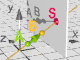10.6 Hit a plane || yz-plane Calculation and visualization of the intersection of a straight line with a plane parallel to the yz-plane.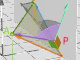10.7 Angle, triangle yz-plane Calculation and visualization of the angles between a triangle surface and the yz-plane.

top
 © Friedrich A. Lohmüller, 2015email: http://www.f-lohmueller.de Visitors:(since May-1999) Thanks for your visit!